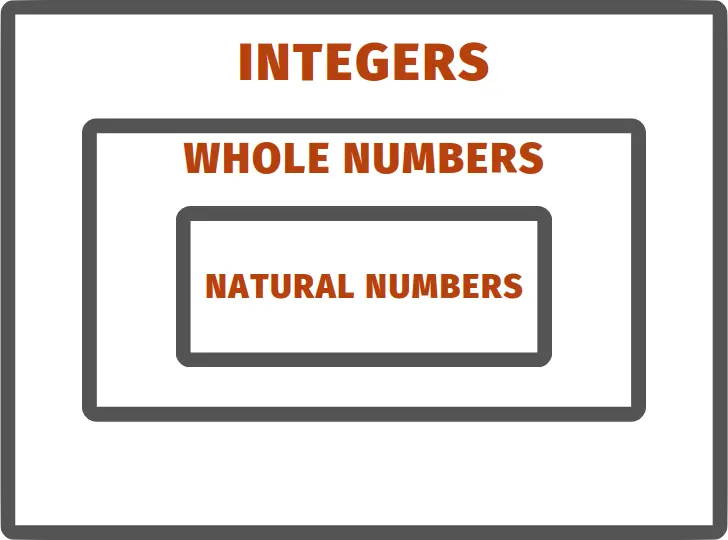# Introduction to Number Theory

In Competitive Programming

## What is Number Theory

Number Theory is the study and its application to solve problems related to integers and integral values functions. Number Theory in Competitive Programming includes, but is not limited to, study and application of

1. Operations like Multiplication, Exponents and Remainders of numbers
2. Factors of Number ( GCD, LCM and Prime numbers )
3. Factorial of Number
4. Permutations and Combinations
5. Sequences of integers, like Fibonacci Series, Prime numbers, square numbers etc.

## Types of Integers

### 1. On The Basis of range of Values

• Positive Integers : These are natural numbers. They include all the numbers from 1 to infinity. So, Positive Integers are 1, 2, 3, 4, ...

• Non-Negative Integers: These are whole numbers. They include all the positive integers and 0. So, Non Negative numbers are 0, 1, 2, 3, 4, ...

• Integers : These include all the positive and negative numbers. So, Integers include ... -4, -3, -2, -1, 0, 1, 2, 3, 4, ...### 2. On the Basis of Series

• Perfect Squares : These are the numbers that are squares of other integers. All perfect squares must be whole numbers. These numbers are 0, 1, 4, 9, 16, ...
• Fibonacci Numbers : These are the numbers from fibonacci series. In this, next numbers are sum of previous 2 numbers. All fibonacci numbers are natural numbers. These numbers are 1, 1, 2, 3, 5, 8, 13, ...
• Perfect Cubes : These are the numbers that are cubes of other integers. These can be positive negative or zero. For example ... -27, -8, -1, 0, 1, 8, 27, ...